# Foldr and Foldl CS 5010 Program Design Paradigms

• Slides: 26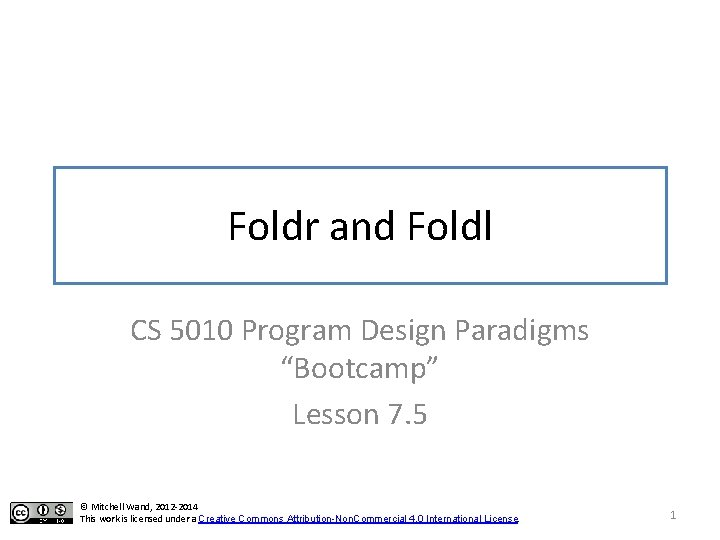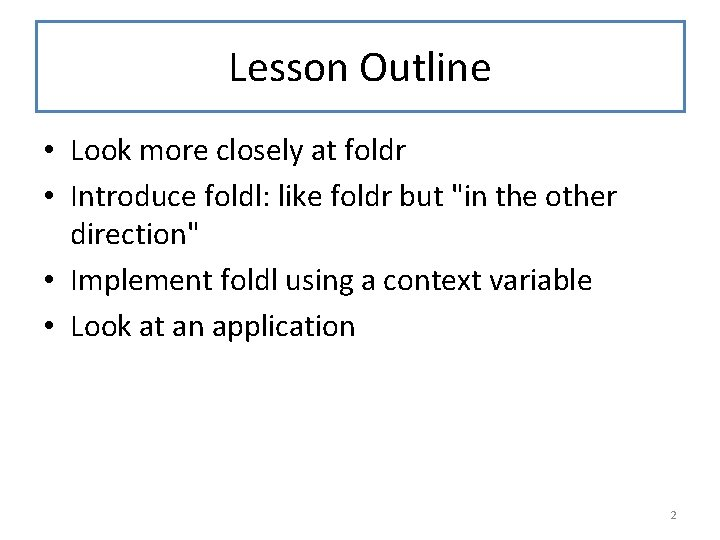Lesson Outline • Look more closely at foldr • Introduce foldl: like foldr but "in the other direction" • Implement foldl using a context variable • Look at an application 2Learning Objectives • At the end of this lesson you should be able to: – explain what foldr and foldl compute – explain the difference between foldr and foldl – explain why they are called "fold right" and "fold left" – use foldl in a function definition 3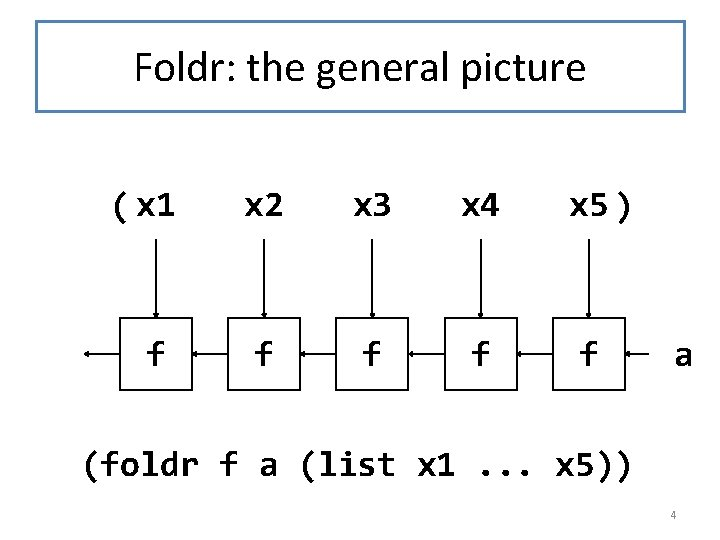Foldr: the general picture ( x 1 x 2 x 3 x 4 x 5 ) f f f a (foldr f a (list x 1. . . x 5)) 4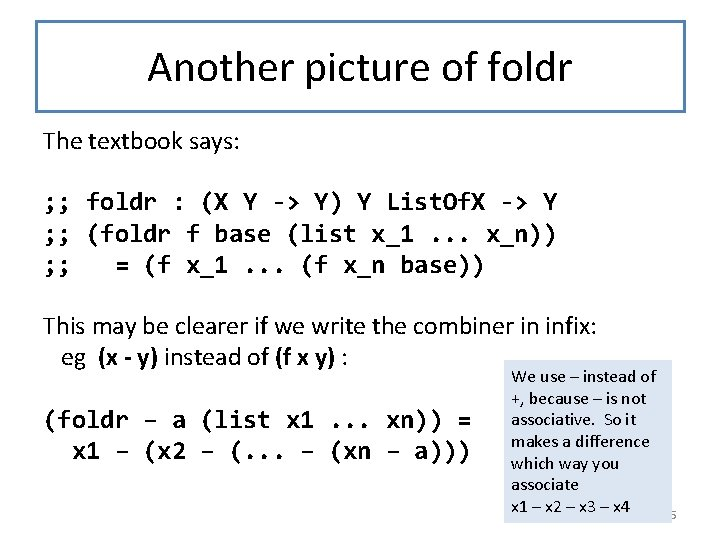Another picture of foldr The textbook says: ; ; foldr : (X Y -> Y) Y List. Of. X -> Y ; ; (foldr f base (list x_1. . . x_n)) ; ; = (f x_1. . . (f x_n base)) This may be clearer if we write the combiner in infix: eg (x - y) instead of (f x y) : (foldr – a (list x 1. . . xn)) = x 1 – (x 2 – (. . . – (xn – a))) We use – instead of +, because – is not associative. So it makes a difference which way you associate x 1 – x 2 – x 3 – x 4 5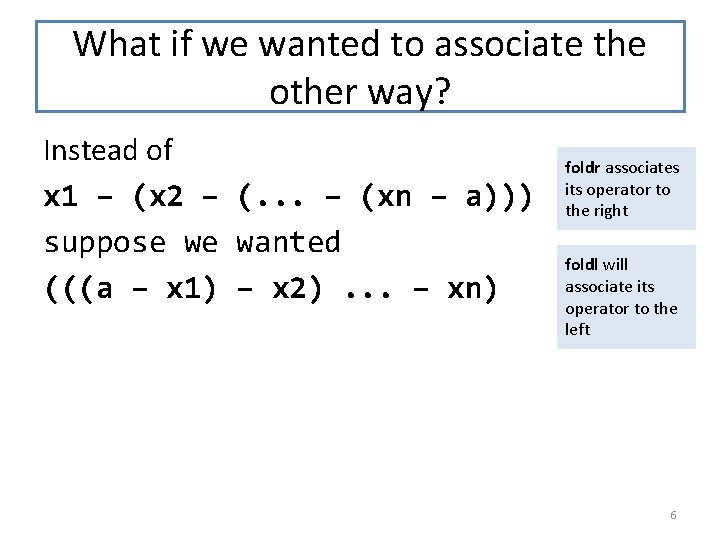What if we wanted to associate the other way? Instead of x 1 – (x 2 – (. . . – (xn – a))) suppose we wanted (((a – x 1) – x 2). . . – xn) foldr associates its operator to the right foldl will associate its operator to the left 6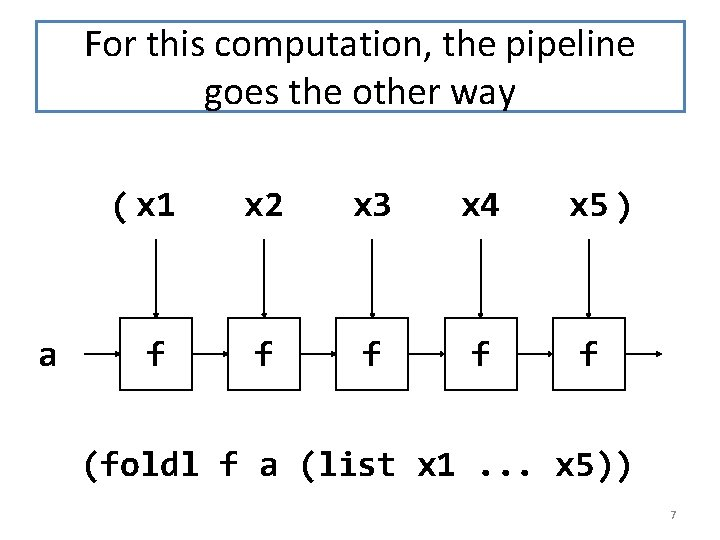For this computation, the pipeline goes the other way a ( x 1 x 2 x 3 x 4 x 5 ) f f f (foldl f a (list x 1. . . x 5)) 7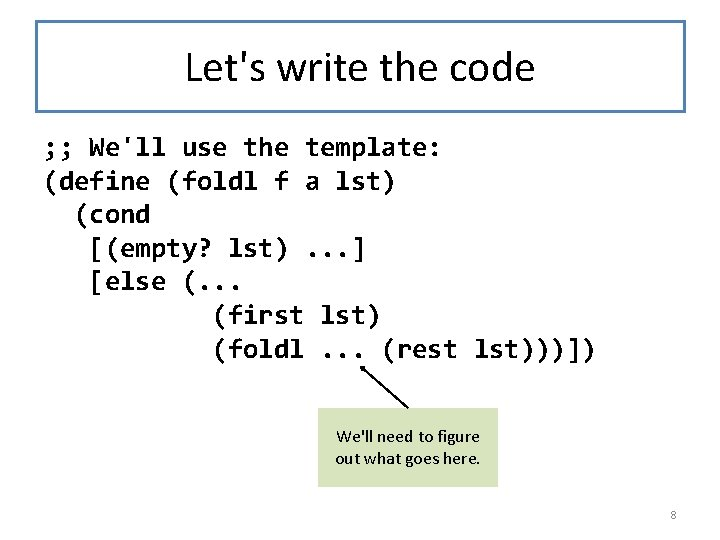Let's write the code ; ; We'll use the template: (define (foldl f a lst) (cond [(empty? lst). . . ] [else (. . . (first lst) (foldl. . . (rest lst)))]) We'll need to figure out what goes here. 8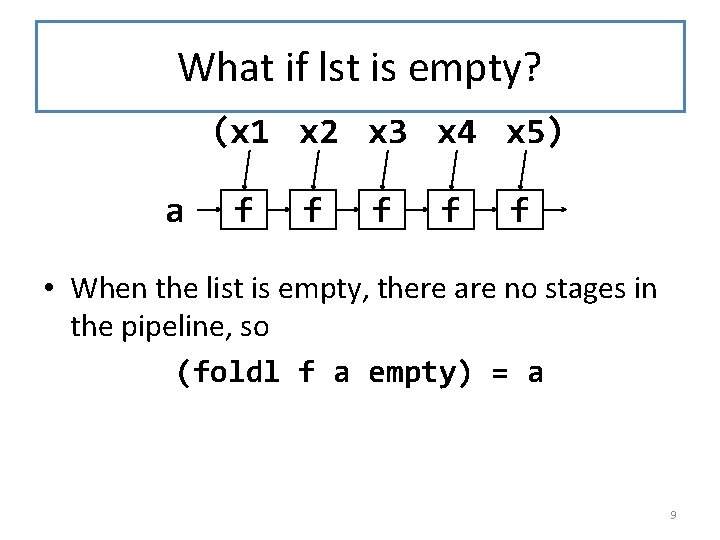What if lst is empty? (x 1 x 2 x 3 x 4 x 5) a f f f • When the list is empty, there are no stages in the pipeline, so (foldl f a empty) = a 9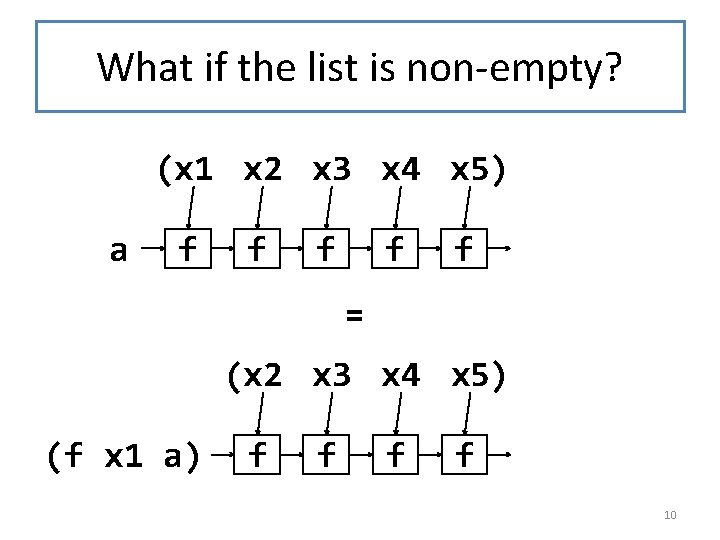What if the list is non-empty? (x 1 x 2 x 3 x 4 x 5) a f f f = (x 2 x 3 x 4 x 5) (f x 1 a) f f 10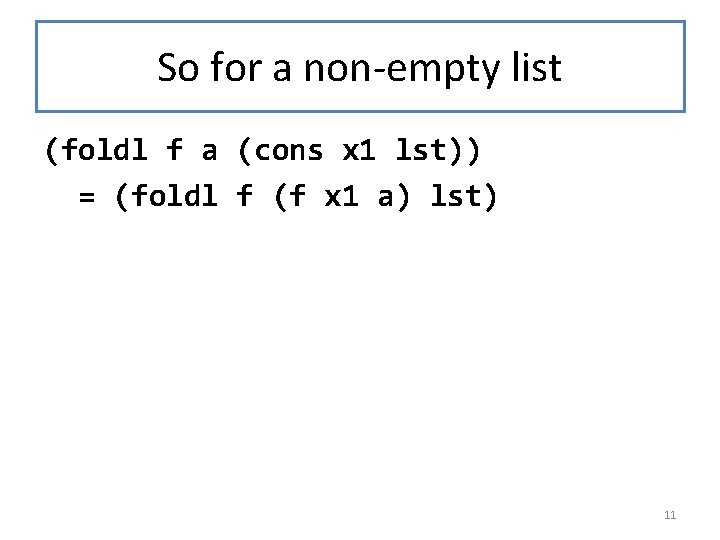So for a non-empty list (foldl f a (cons x 1 lst)) = (foldl f (f x 1 a) lst) 11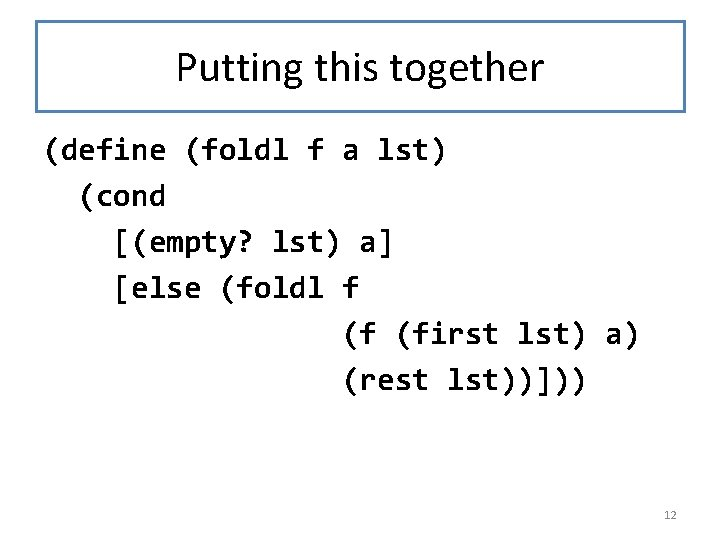Putting this together (define (foldl f a lst) (cond [(empty? lst) a] [else (foldl f (f (first lst) a) (rest lst))])) 12Let's do a computation (foldl - 1 (list 20 10 2)) = (foldl - 19 (list 10 2)) ; 20 -1 = 19 = (foldl - -9 (list 2)) ; 10 -19 = -9 = (foldl - 11 empty) ; 2 -(-9) = 11 13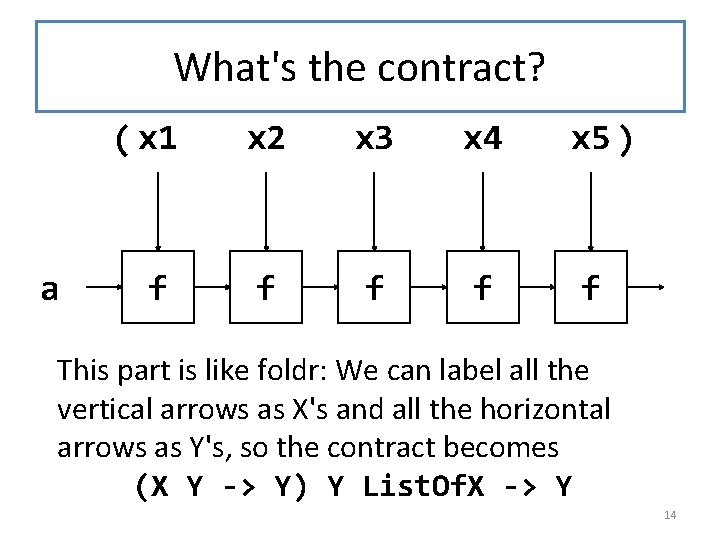What's the contract? a ( x 1 x 2 x 3 x 4 x 5 ) f f f This part is like foldr: We can label all the vertical arrows as X's and all the horizontal arrows as Y's, so the contract becomes (X Y -> Y) Y List. Of. X -> Y 14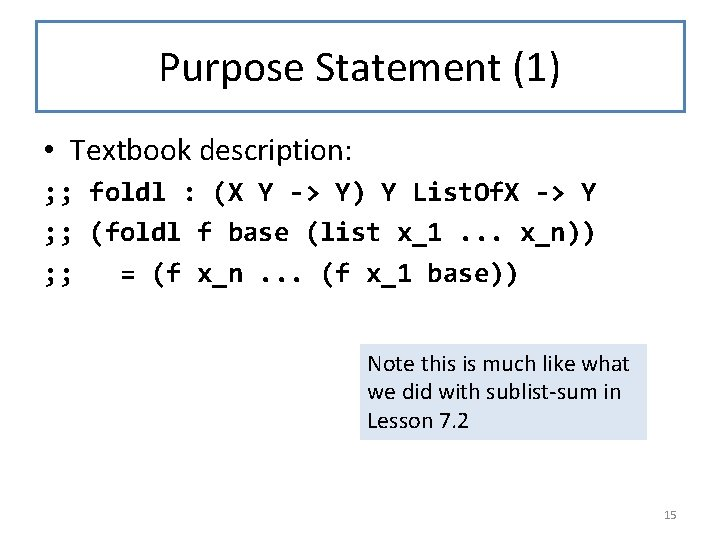Purpose Statement (1) • Textbook description: ; ; foldl : (X Y -> Y) Y List. Of. X -> Y ; ; (foldl f base (list x_1. . . x_n)) ; ; = (f x_n. . . (f x_1 base)) Note this is much like what we did with sublist-sum in Lesson 7. 2 15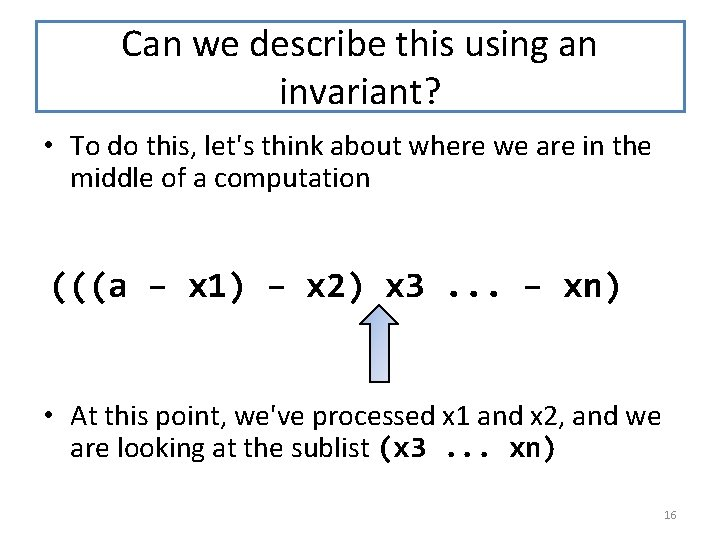Can we describe this using an invariant? • To do this, let's think about where we are in the middle of a computation (((a – x 1) – x 2) x 3. . . – xn) • At this point, we've processed x 1 and x 2, and we are looking at the sublist (x 3. . . xn) 16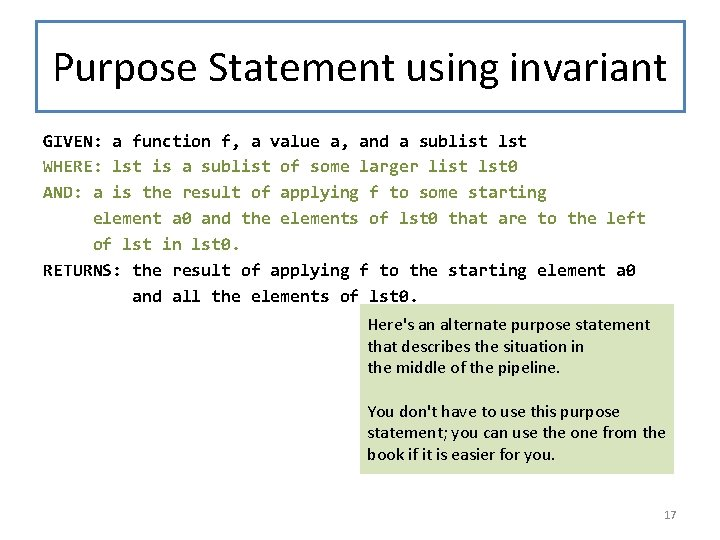Purpose Statement using invariant GIVEN: a function f, a value a, and a sublist lst WHERE: lst is a sublist of some larger list lst 0 AND: a is the result of applying f to some starting element a 0 and the elements of lst 0 that are to the left of lst in lst 0. RETURNS: the result of applying f to the starting element a 0 and all the elements of lst 0. Here's an alternate purpose statement that describes the situation in the middle of the pipeline. You don't have to use this purpose statement; you can use the one from the book if it is easier for you. 17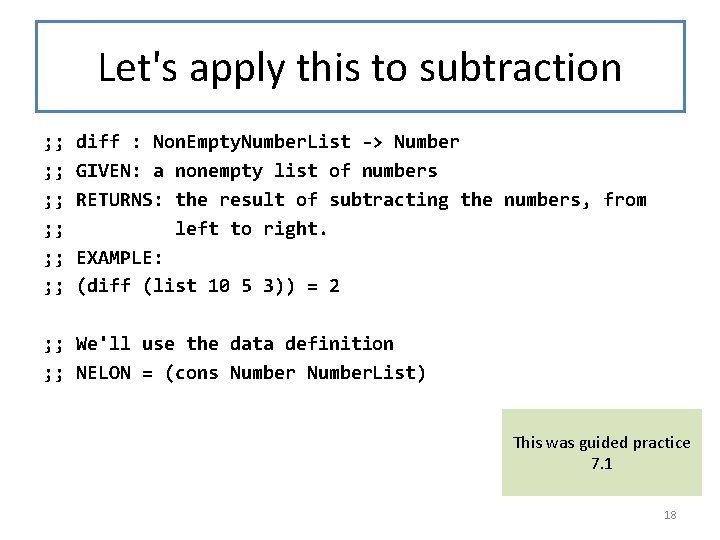Let's apply this to subtraction ; ; diff : Non. Empty. Number. List -> Number ; ; GIVEN: a nonempty list of numbers ; ; RETURNS: the result of subtracting the numbers, from ; ; left to right. ; ; EXAMPLE: ; ; (diff (list 10 5 3)) = 2 ; ; We'll use the data definition ; ; NELON = (cons Number. List) This was guided practice 7. 1 18Code, with simple purpose statement (define (diff nelst) (diff-inner (first nelst) (rest nelst))) ; ; diff-inner : Number. List ; ; RETURNS: the result of subtracting each of the numbers in lon ; ; from num (define (diff-inner num lon) (cond [(empty? lon) num] [else (diff-inner (- num (first lon)) ; ; this is (f a (first lon)) ; ; different order of arguments ; ; than foldl (rest lon))])) 19Code, with fancier purpose statement (define (diff nelst) (diff-inner (first nelst) (rest nelst))) sofar is a good name for this argument ; ; diff-inner : Number. List ; ; GIVEN: a number sofar and a sublist lon of some list lon 0 ; ; WHERE: sofar is the result of subtracting all the numbers in ; ; lon 0 that are above lon. ; ; RETURNS: the result of subtracting all the numbers in lon 0. (define (diff-inner sofar lon) (cond [(empty? lon) sofar] [else (diff-inner (- sofar (first lon)) ; ; this is (f a (first lon)) ; ; different order of arguments ; ; than foldl (rest lon))])) You could use either this purpose statement or the on the preceding slide. Both are fine. 20Or using foldl (define (diff nelst) (foldl (lambda (x sofar) (- sofar x)) ; ; foldl wants an X Y -> Y (first nelst) (rest nelst))) sofar is a good name for this argument, because it describes where the value comes from. 21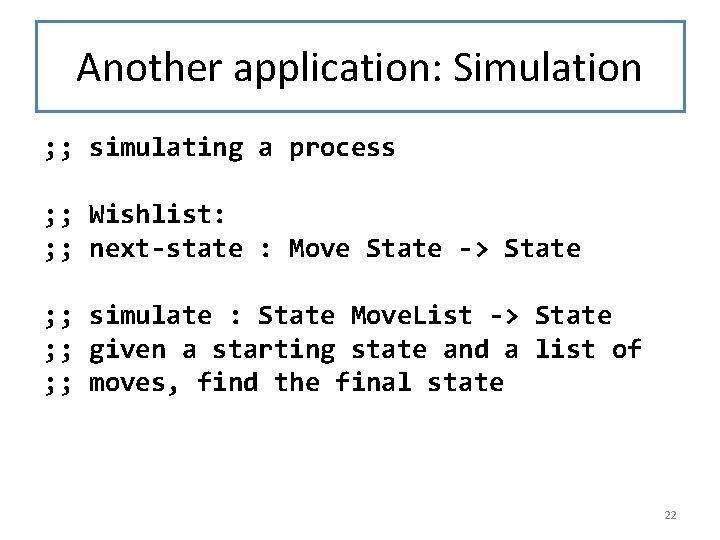Another application: Simulation ; ; simulating a process ; ; Wishlist: ; ; next-state : Move State -> State ; ; simulate : State Move. List -> State ; ; given a starting state and a list of ; ; moves, find the final state 22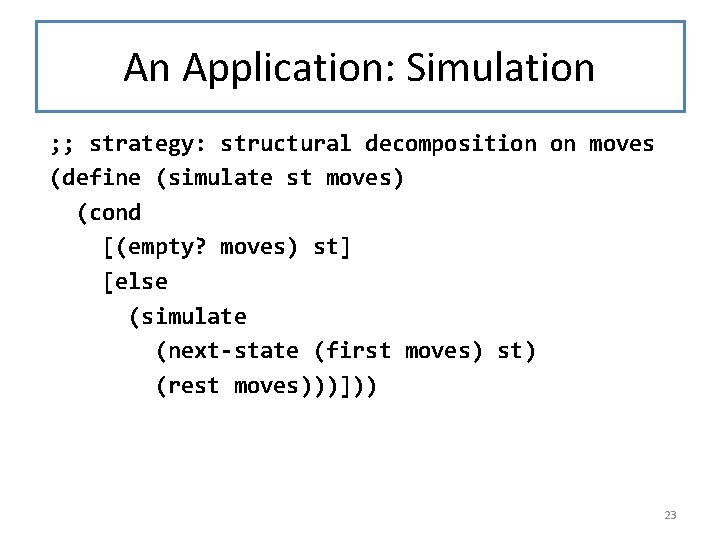An Application: Simulation ; ; strategy: structural decomposition on moves (define (simulate st moves) (cond [(empty? moves) st] [else (simulate (next-state (first moves) st) (rest moves)))])) 23Or using foldl (define (simulate initial-state moves) (foldl I carefully chose the order of the arguments to make this work. If next-state took its arguments in a different order, you'd have to do initial-state the same kind of thing we did for moves)) subtraction above. 24Summary • You should now be able to: – explain what foldr and foldl compute – explain the difference between foldr and foldl – explain why they are called "fold right" and "fold left" – use foldl in a function definition 25